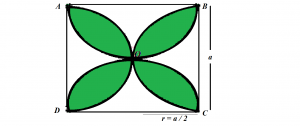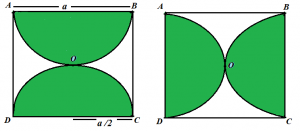Related Articles

# Find the area of the shaded region formed by the intersection of four semicircles in a square

• Difficulty Level : Medium
• Last Updated : 19 Apr, 2021

Given the length of the side of a square a, the task is to find the area of the shaded region formed by the intersection of four semicircles in a square as shown in the image below:Examples:

Input: a = 10
Output: 57
Input: a = 19
Output: 205.77

Approach: Area of the shaded region will be:

Area(semicircle1) + Area(semicircle2) + Area(semicircle3) + Area(semicircle4) – Area(square).
Since all semicircles are of same radius, therefore, area of all semicircles will be equal. So, the above formula can be written as:
4*(Area of Semicircle) – Area(Square)

The area of a semicircle is (3.14 * r2) / 2 where r is the radius of the semicircle which is equal to a / 2.
Hence, Area of the shaded region = 4 * (3.14 * (a * a) / 8 ) – a * aBelow is the implementation of the above approach:

## C++

 `// C++ implementation of the approach``#include ``using` `namespace` `std;` `// Function to return the area``// of the shaded region``float` `findAreaShaded(``float` `a)``{` `    ``// Area of the square``    ``float` `sqArea = a * a;` `    ``// Area of the semicircle``    ``float` `semiCircleArea = (3.14 * (a * a) / 8);` `    ``// There are 4 semicircles``    ``// shadedArea = Area of 4 semicircles - Area of square``    ``float` `ShadedArea = 4 * semiCircleArea - sqArea;` `    ``return` `ShadedArea;``}` `// Driver code``int` `main()``{``    ``float` `a = 10;``    ``cout << findAreaShaded(a);` `    ``return` `0;``}`

## Java

 `// Java implementation of the approach``class` `GFG {` `    ``// Function to return the area``    ``// of the shaded region``    ``static` `float` `findAreaShaded(``float` `a)``    ``{` `        ``// Area of the square``        ``float` `sqArea = a * a;` `        ``// Area of the semicircle``        ``float` `semiCircleArea = (``float``)(``3.14` `* (a * a) / ``8``);` `        ``// There are 4 semicircles``        ``// shadedArea = Area of 4 semicircles - Area of square``        ``float` `ShadedArea = ``4` `* semiCircleArea - sqArea;` `        ``return` `ShadedArea;``    ``}` `    ``// Driver code``    ``public` `static` `void` `main(String[] args)``    ``{``        ``float` `a = ``10``;``        ``System.out.println(findAreaShaded(a));``    ``}``}`

## Python3

 `# Python3 implementation of the approach` `# Function to return the area``# of the shaded region``def` `findAreaShaded(a):``    ` `    ``# Area of the square``    ``sqArea ``=` `a ``*` `a;` `    ``# Area of the semicircle``    ``semiCircleArea ``=` `(``3.14` `*` `(a ``*` `a ) ``/` `8``)` `    ``# There are 4 semicircles``    ``# shadedArea = Area of 4 semicircles - Area of square``    ``ShadedArea ``=` `4` `*` `semiCircleArea ``-` `sqArea ;` `    ``return` `ShadedArea;` `# Driver code``if` `__name__ ``=``=` `'__main__'``:``    ``a ``=` `10``    ``print``(findAreaShaded(a))`

## C#

 `// C# implementation of the approach``using` `System;` `class` `GFG``{` `    ``// Function to return the area``    ``// of the shaded region``    ``static` `float` `findAreaShaded(``float` `a)``    ``{` `        ``// Area of the square``        ``float` `sqArea = a * a;` `        ``// Area of the semicircle``        ``float` `semiCircleArea = (``float``)(3.14 * (a * a) / 8);` `        ``// There are 4 semicircles``        ``// shadedArea = Area of 4 semicircles - Area of square``        ``float` `ShadedArea = 4 * semiCircleArea - sqArea;` `        ``return` `ShadedArea;``    ``}` `    ``// Driver code``    ``public` `static` `void` `Main()``    ``{``        ``float` `a = 10;``        ``Console.WriteLine(findAreaShaded(a));``    ``}``}` `// This code is contributed by mohit kumar 29`

## PHP

 ``

## Javascript

 ``
Output:
`57`

Attention reader! Don’t stop learning now. Participate in the Scholorship Test for First-Step-to-DSA Course for Class 9 to 12 students.

My Personal Notes arrow_drop_up